Courses

# Statistical Physics MCQ Level – 1

## 10 Questions MCQ Test Topic wise Tests for IIT JAM Physics | Statistical Physics MCQ Level – 1

Description
This mock test of Statistical Physics MCQ Level – 1 for Physics helps you for every Physics entrance exam. This contains 10 Multiple Choice Questions for Physics Statistical Physics MCQ Level – 1 (mcq) to study with solutions a complete question bank. The solved questions answers in this Statistical Physics MCQ Level – 1 quiz give you a good mix of easy questions and tough questions. Physics students definitely take this Statistical Physics MCQ Level – 1 exercise for a better result in the exam. You can find other Statistical Physics MCQ Level – 1 extra questions, long questions & short questions for Physics on EduRev as well by searching above.
QUESTION: 1

### In the equilibrium state, the thermodynamic probability of a system is. Select one:

Solution:

In the equilibrium state, the system attains the most stable state and the only possibility of finding the system is that particular equilibrium state, hence the probability in that state is 1.

QUESTION: 2

### A monoatomic crystalline solid comprises of N atoms, out of which n atoms are in interstitial positions. If the available interstitial sites are N', then number of possible microstates is. Select one:

Solution:

According to probability theory,
Number of ways for choosing n atoms out of N atoms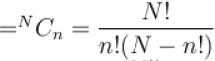and number of ways for putting n atoms in N' site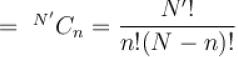∴ Total number of microstates are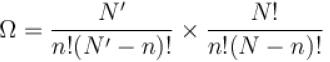The correct answer is: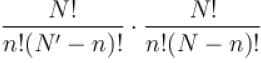QUESTION: 3

### Thermodynamic variables of a system can be volume V, pressure p, temperature T, number of particular N, internal energy E and chemical potential µ etc. For a system to be specified by microcanonical (MC), canonical ensemble (CE) and Grand Canonical (GC) ensembles, the parameters required for the respective example are :Select one:

Solution:

Micro canonical ensemble, N, V and E are constants of the ensemble, hence MC : (E, V, N)
For canonical ensemble N, V and T are constants hence CE : (N, V, T)
For grand canonical ensemble, V, T and µ are constants hence GC : (V, T, µ)
The correct answer is: MC : (E, V, N); CE : (N, V, T); GC : (V, T, µ)

QUESTION: 4

If ρ represents the density of phase space. Which of the following is the equation for Liouville’s theorem.
Select one:

Solution:

It is about conservation of density of point  in phase space.
∴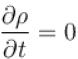or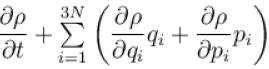Using Hamiltonian’s equation of motion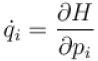and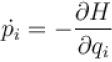We get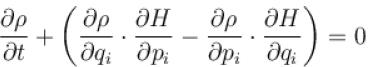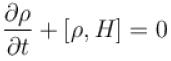[ρ,H] represents poisson’s bracket.
The correct answer is: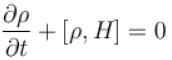QUESTION: 5

In a microcanonical ensemble, a system A of fixed volume is in contact with a large reservoir B. Then
Select one:

Solution:

In a microcanonical ensemble, the system is assumed to be isolated in the sense that the system cannot exchange energy or particles with its environment, so that the energy of the system remains exactly known as the time goes on.
The correct answer is: A can exchange neither energy nor particles with B

QUESTION: 6

Canonical ensemble is related to.
Select one:

Solution:

A canonical ensemble is the statistical ensemble that represents the possible states of a mechanical system in thermal equilibrium with a heat bath at a fixed temperature.
The correct answer is: Thermal equilibrium of system

QUESTION: 7

In a classical microcanonical ensemble for a system of N interacting particles, the fundamental volume in phase space which is regarded as equivalent to one microstate is.
Select one:

Solution:

For the particle, volume of phase space corresponding to one microstate is
dV = (dxdydz)(dpxdpydpz)
= h3
For N interacting particles, the volume of one microstate is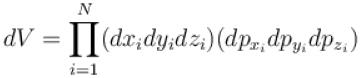= (h3)·h3 ···N terms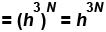QUESTION: 8

A one-dimensional random walker takes steps to the left or right with equal probability. The probability that the random walker starting from the origin is back to the origin after N even number of steps is.
Select one:

Solution:

Probability is given by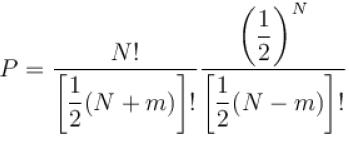Here m = 0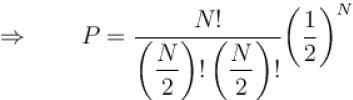The correct answer is: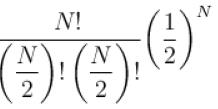QUESTION: 9

In a canonical ensemble, a system A of fixed volume is in contact with a large reservoir B then
Select one:

Solution:

In canonical ensemble, system can exchange energy with the heat bath so that the states of the system will differ in total energy.
The correct answer is: A can exchange only energy with B

QUESTION: 10

For a microcanonical ensemble, the phase density inside a small energy interval remains constant and outside such a region, it is.
Select one:

Solution:

Since, we take energy interval where phase density remains constant, and energy is the constant of the ensemble, we tend to consider the phase density to be zero outside thus interval.